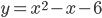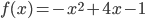### Day 36 - Graphing Quadratic Functions - 03.04.15

• Video Protocol - WNQ ("wink")
• Summative Exam 1 this Friday, March 6th
• Schedule
• Wednesday - Review/New Material
• Thursday - Review/New Material
• Friday - Summative Exam 1

Questions

Bell Ringer

1. What is the graph of a quadratic function called?

2. parabol

3. curved line

4. parabola

5. none of the above

2. What term must every quadratic function have?

1. x

2. x2

3. c

4. b

5. none of the above

3. What is the equation of the axis of symmetry for the following function:1. x = -1/2

2. x = 1/2

3. x = 1

4. x = -6

5. none of the above

4. What are the coordinates of the vertex for the following function:1. (2,3)

2. (-2,3)

3. (2,-3)

4. (-2,-3)

5. none of the above

5. What type of form is the following quadratic function in?

1. standard

2. vertex

3. factored

5. none of the above

Review
• N/A

Lesson
2. Recognize that a quadratic function has the shape of a parabola.
3. Recognize standard form of a quadratic function.
4. Recognize factored form of a quadratic function.
5. Recognize vertex form of a quadratic function.
6. Find the coordinates of the vertex of a parabola.
7. Find the equation of the axis of symmetry.
8. Find the minimum or maximum of a quadratic function.

Exit Ticket
• Posted on the board at the end of the block
Lesson Objective(s)
• How are key features of a graph of quadratic functions found?
Skills
2. Recognize that a quadratic function has the shape of a parabola.
3. Recognize standard form of a quadratic function.
4. Recognize factored form of a quadratic function.
5. Recognize vertex form of a quadratic function.
6. Find the coordinates of the vertex of a parabola.
7. Find the equation of the axis of symmetry.
8. Find the minimum or maximum of a quadratic function.
9. Find the x and y-intercepts of a quadratic function.

#### In-Class Help Requests

Standard(s)
• N/A

Mathematical Practice(s)
• #1 - Make sense of problems and persevere in solving them
• #2 - Reason abstractly and quantitatively
• #7 - Look for and make use of structure

Past Checkpoints
• N/A